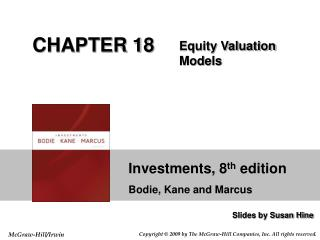Download PresentationCHAPTER 18CHAPTER 18 - PowerPoint PPT Presentation

Download PresentationCHAPTER 18
An Image/Link below is provided (as is) to download presentation

Download Policy: Content on the Website is provided to you AS IS for your information and personal use and may not be sold / licensed / shared on other websites without getting consent from its author. While downloading, if for some reason you are not able to download a presentation, the publisher may have deleted the file from their server.

- - - - - - - - - - - - - - - - - - - - - - - - - - - E N D - - - - - - - - - - - - - - - - - - - - - - - - - - -
Presentation Transcript

1. CHAPTER 18 Equity Valuation Models

2. Balance Sheet Models Book Value Dividend Discount Models Price/Earning Ratios Models of Equity Valuation

3. Table 18.1 Financial Highlights for Microsoft Corporation, October 25, 2007

4. Limitations of Book Value Book value is an application of arbitrary accounting rules Can book value represent a floor value? Better approaches Liquidation value Replacement cost

5. Expected Holding Period Return The return on a stock investment comprises cash dividends and capital gains or losses Assuming a one-year holding period

6. Required Return CAPM gave us required return: If the stock is priced correctly Required return should equal expected return

7. Intrinsic Value Self assigned Value Variety of models are used for estimation Market Price Consensus value of all potential traders Trading Signal IV > MP Buy IV < MP Sell or Short Sell IV = MP Hold or Fairly Priced Intrinsic Value and Market Price

8. PH = the expected sales price for the stock at time H H = the specified number of years the stock is expected to be held Specified Holding Period

9. V0 = Value of Stock Dt= Dividend k = required return Dividend Discount Models: General Model

10. Stocks that have earnings and dividends that are expected to remain constant Preferred Stock No Growth Model

11. E1 = D1 = \$5.00 k = .15 V0 = \$5.00 /.15 = \$33.33 No Growth Model: Example

12. g = constant perpetual growth rate Constant Growth Model

13. E1 = \$5.00 b = 40% k = 15% (1-b) = 60% D1= \$3.00 g = 8% V0 = 3.00 / (.15 - .08) = \$42.86 Constant Growth Model: Example

14. g = growth rate in dividends ROE = Return on Equity for the firm b = plowback or retention percentage rate (1- dividend payout percentage rate) Estimating Dividend Growth Rates

15. Present Value of Growth Opportunities If the stock price equals its IV, growth rate is sustained, the stock should sell at: If all earnings paid out as dividends, price should be lower (assuming growth opportunities exist)

16. Present Value of Growth Opportunities Continued Price = No-growth value per share + PVGO (present value of growth opportunities)

17. ROE = 20% d = 60% b = 40% E1 = \$5.00 D1 = \$3.00 k = 15% g = .20 x .40 = .08 or 8% Partitioning Value: Example

18. Partitioning Value: Example Continued Vo = value with growth NGVo = no growth component value PVGO = Present Value of Growth Opportunities

19. Life Cycles and Multistage Growth Models g1 = first growth rate g2 = second growth rate T = number of periods of growth at g1

20. Multistage Growth Rate Model: Example D0 = \$2.00 g1= 20% g2= 5% k = 15% T = 3 D1= 2.40 D2 = 2.88 D3= 3.46 D4= 3.63 V0= D1/(1.15) + D2/(1.15)2 + D3/(1.15)3 + D4 / (.15 - .05) ( (1.15)3 V0= 2.09 + 2.18 + 2.27 + 23.86 = \$30.40

21. Table 18.2 Financial Ratios in Two Industries

22. P/E Ratios are a function of two factors Required Rates of Return (k) Expected growth in Dividends Uses Relative valuation Extensive Use in industry Price Earnings Ratios

23. E1 - expected earnings for next year E1 is equal to D1 under no growth k - required rate of return P/E Ratio: No Expected Growth

24. b = retention ratio ROE = Return on Equity P/E Ratio: Constant Growth

25. E0 = \$2.50 g = 0 k = 12.5% P0 = D/k = \$2.50/.125 = \$20.00 PE = 1/k = 1/.125 = 8 Numerical Example: No Growth

26. b = 60% ROE = 15% (1-b) = 40% E1= \$2.50 (1 + (.6)(.15)) = \$2.73 D1 = \$2.73 (1-.6) = \$1.09 k = 12.5% g = 9% P0 = 1.09/(.125-.09) = \$31.14 PE = 31.14/2.73 = 11.4 PE = (1 - .60) / (.125 - .09) = 11.4 Numerical Example: Growth

27. P/E Ratios and Stock Risk Holding all else equal Riskier stocks will have lower P/E multiples Higher values of k; therefore, the P/E multiple will be lower

28. Pitfalls in P/E Analysis Use of accounting earnings Earnings Management Choices on GAAP Inflation Reported earnings fluctuate around the business cycle

29. Figure 18.3 P/E Ratios of the S&P 500 Index and Inflation

30. Figure 18.4 Earnings Growth for Two Companies

31. Figure 18.5 Price-Earnings Ratios

32. Figure 18.6 P/E Ratios for Different Industries, 2007

33. Other Comparative Value Approaches Price-to-book ratio Price-to-cash-flow ratio Price-to-sales ratio

34. Figure 18.7 Market Valuation Statistics

35. Free Cash Flow Approach Discount the free cash flow for the firm Discount rate is the firm’s cost of capital Components of free cash flow After tax EBIT Depreciation Capital expenditures Increase in net working capital

36. Comparing the Valuation Models In practice Values from these models may differ Analysts are always forced to make simplifying assumptions

37. The Aggregate Stock Market Explaining Past Behavior Forecasting the Stock Market

38. Figure 18.8 Earnings Yield of S&P 500 versus 10-Year Treasury-Bond Yield

39. Table 18.4 S&P 500 Price Forecasts Under Various Scenarios